# Important Questions Test: Table Charts- 2

## 5 Questions MCQ Test IBPS Clerk Prelims - Study Material, Mock Tests | Important Questions Test: Table Charts- 2

Description
Attempt Important Questions Test: Table Charts- 2 | 5 questions in 7 minutes | Mock test for Banking Exams preparation | Free important questions MCQ to study IBPS Clerk Prelims - Study Material, Mock Tests for Banking Exams Exam | Download free PDF with solutions
QUESTION: 1

### Study the following table and answer the questions based on it.What is the average amount of interest per year which the company had to pay during this period?

Solution:

Average amount of interest paid by the Company during the given period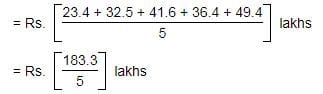= Rs. 36.66 lakhs.

QUESTION: 2

### Study the following table and answer the questions based on it.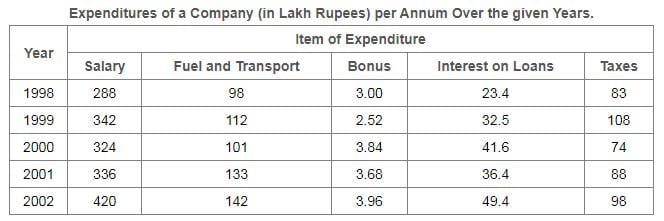The total amount of bonus paid by the company during the given period is approximately what percent of the total amount of salary paid during this period?

Solution:

[(300 + 2.52 + 3.84 + 3.68 + 3.96)*100]/[288 + 342 + 324 + 336 + 420]
= (17/1710)*100
which is approximately equal to 1.

QUESTION: 3

### Study the following table and answer the questions based on it.Total expenditure on all these items in 1998 was approximately what percent of the total expenditure in 2002?

Solution: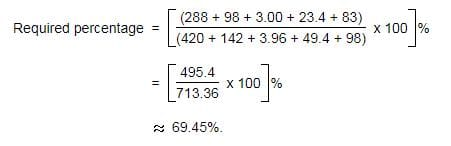QUESTION: 4

Study the following table and answer the questions based on it.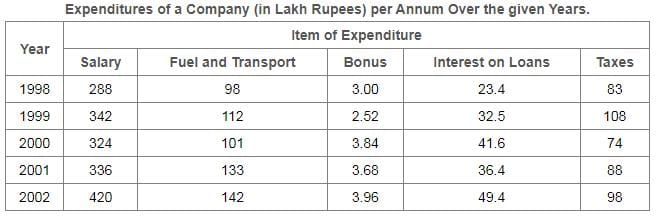The total expenditure of the company over these items during the year 2000 is?

Solution:

Total expenditure of the Company during 2000
= Rs. (324 + 101 + 3.84 + 41.6 + 74)lakhs
= Rs. 544.44 lakhs.

QUESTION: 5

Study the following table and answer the questions based on it.The ratio between the total expenditure on Taxes for all the years and the total expenditure on Fuel and Transport for all the years respectively is approximately?

Solution: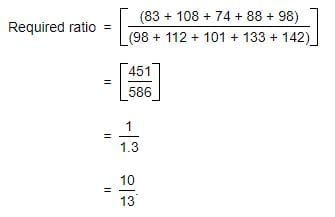Use Code STAYHOME200 and get INR 200 additional OFF Use Coupon Code